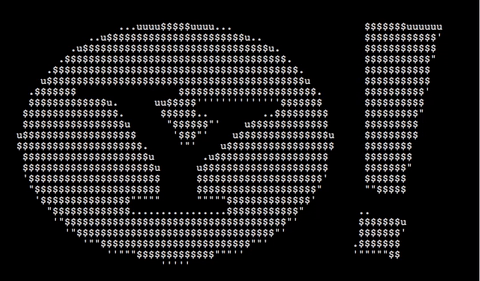`````` 1 2 3 4 5 6 7 8 9 10 11 12 13 14 15 16 17 18 19 20 21 22 23 24 25 26 27 28 `````` ``````#include #include #include int c,p,i,j,n,F=40,k,m; float a,x,y,S=0,V=0; void main() { for(;F--;usleep(50000),F?puts("x1b[25A"):0) { for(S+=V+=(1-S)/10-V/4,j=0;j<72;j+=3,putchar(10)) { for(i=0;x=S*(i-27),i++<73;putchar(c[" ''".\$u\$"])) { for(c=0,n=3;n--;) { for(y=S*(j+n-36),k=0,c^=(136*x*x+84*y*y<92033)<x*cos(a)+y*sin(a)?k=p,p="S+=V+=(1-S)/10-V/4 是该动画的欠阻尼控制系统——S 是缩放（=1/缩放倍数）、V 是速度、1/10 和 1/4 是 PD 常量。在第一帧中 S=0 相当于无穷放大。S<0 是非法的。F 是帧数计数器。1.16 控制多边形绘制的缩放比例（68 是 79/1.16 的近似值，所以也需要对它进行校正），而 136、84、92033 定义了一个椭圆。14.64 是一个不可调参数（它是 46/π 的值）。(a,b) 构成线段的正交向量。它们指向多边形的内部，所以我们可以直接从定义线段的点得到 a 和 b，即将定义一条边的向量 (x1 – x0, y1 – y0) 旋转 90 度，得到 (a, b) = (y1 – y0, x0 – x1)。然后，由于实际的大小不重要，所以我们对 (a,b) 进行归一化。当我们求解角度时，它们会变成 1。我们在后面可以抵消 c 的取值（假设 ax+by>c，那么对于缩放倍数 s>0，可以得到 sax+sby>sc）。接着计算 θ = atan2(a,b)，将其量化成我们 94 个角度中的一个，并得到 (a,b) = (cos(θ), sin(θ))。